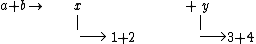# Goldhaber triangle

## Goldhaber triangle

The phase space triangle, or Goldhaber triangle, corresponds to the kinematically allowed boundary for a high-energy reaction leading to four or more particles. In a high-energy reaction between two particles a and b yielding four particles 1, 2, 3, and 4 in the final state (a + b → 1 + 2 + 3 + 4), it is convenient to consider the reaction in terms of the production of two intermediate-state quasiparticle composites x and y, which then decay into two particles each, as in expression (1).

(1)Most high-energy interactions indeed proceed through such intermediate steps, in which, for specific values of the invariant masses mx = Mx* and my = My*, the quasi-particle composites may form resonances. However, the description in terms of the composites x and y, with the invariant masses mx and my as variables, is valid irrespective of whether or not these composites form resonances.

The kinematical limits in this representation are particularly simple, namely, they form a right-angle isosceles triangle. A Goldhaber triangle plot corresponds to plotting a point (mx, my) for each event occurring in the above high-energy reaction. Because of the kinematical constraints, these points must all lie inside the triangle.

If one considers the general reaction given in Eq. (1), then the length of each of the two equal sides of the triangle is Q, defined in Eq. (2).

(2)Here W is the total energy in the center of mass of particles a and b, and mi, for i = 1 to 4, is the mass of the particles 1 to 4. Hence Q corresponds to the total kinetic energy available in the reaction.

In the triangle corresponding to the general reaction (see illustration), the vertical and horizontal bands indicate resonances at masses Mx* and My* with full width at half-maximum height or Γx and Γy, respectively. The bands shown of width 2Γ represent the regions usually chosen if the events corresponding to a given resonance are selected.

## Goldhaber triangle

[′gōlt‚hä·bər ‚trī‚aŋ·gəl]
(particle physics)
A plot describing a high-energy reaction leading to four or more particles; its coordinates are the invariant masses of two intermediate-state quasi-particle composites, and its kinematical limits form a right-angled isosceles triangle; resonances in the quasi-particle composites appear as horizontal and vertical bands.
Site: Follow: Share:
Open / Close